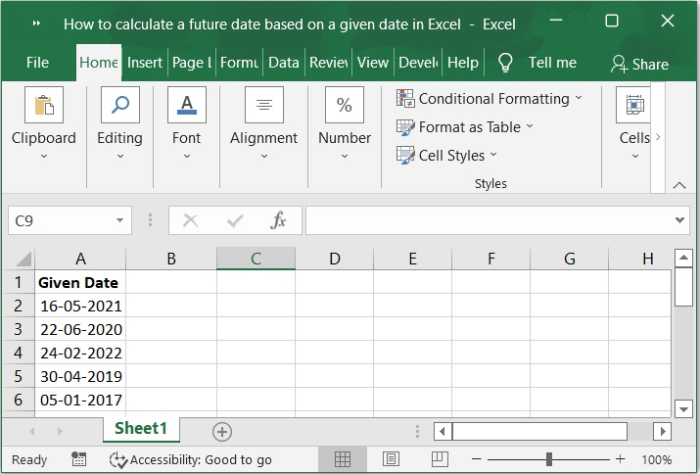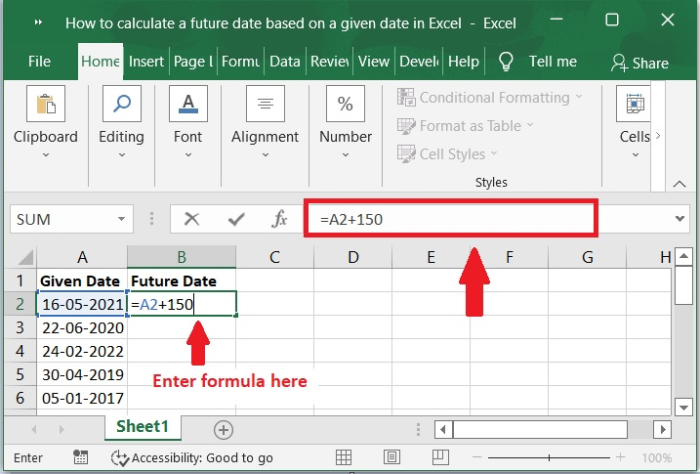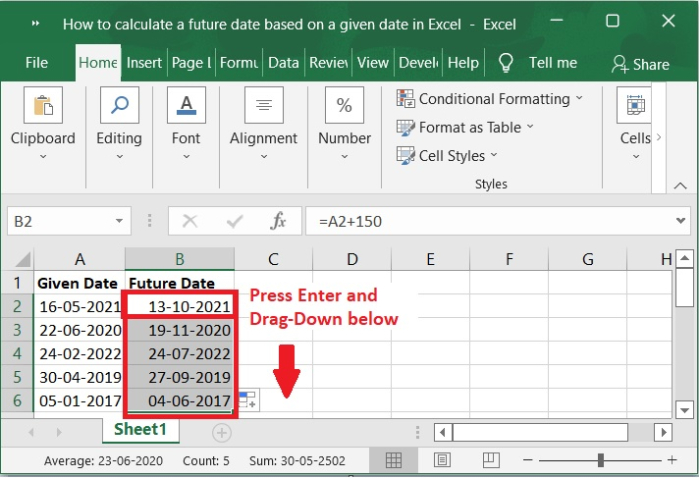# How to calculate a future date based on a given date in Excel?

It is really helpful to perform calculations that involve dates whenever you are dealing with Microsoft Excel. It's possible that you'll need to compute a date in the future in Excel in order to determine when a bill is due or when you might anticipate earning income on an investment. Excel has a number of different date functions that can be used to perform mathematical operations on dates and times.

This tutorial will walk you through the steps of calculating a future date in Excel depending on the data that you provide. In order to determine a date in the future using a formula in Excel, how do I add one or more days to a current date?

Use the following straightforward method to calculate a day in the future that is 150 days after the current date, based on that date.

Let’s understand step by step with an example.

### Step 1

In the first step, let’s assume we have a sample data as shown in the below screenshot.### Step 2

Let's say you have a list of dates in cells A1:A6 and you want to add 150 days to them so that they represent the future. This formula can be entered into a blank cell in column B. Please check out the screenshot below for same.

### Formula

=A2+150### Step 3

After that, you will need to press the enter key on your keyboard and then drag the auto fill handler over the other cells in order to use this formula to add the number 150 and obtain the future dates. Please refer to the below screenshot for the same.## Conclusion

I hope this tutorial helped you understand how to calculate a future date based on a given date by using a simple formula in Excel.